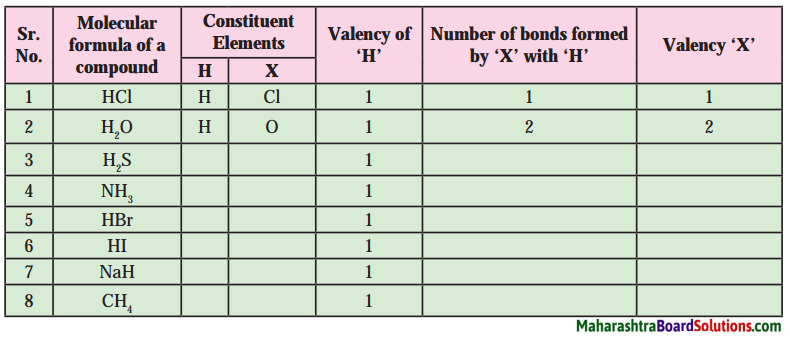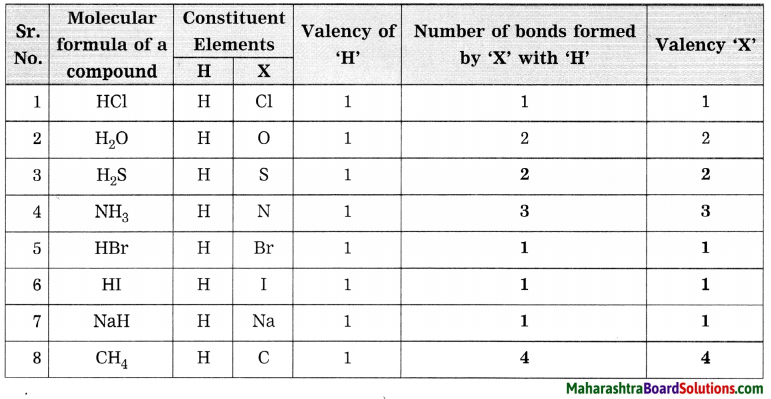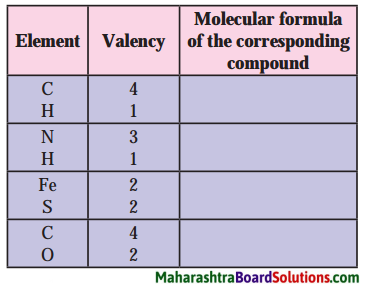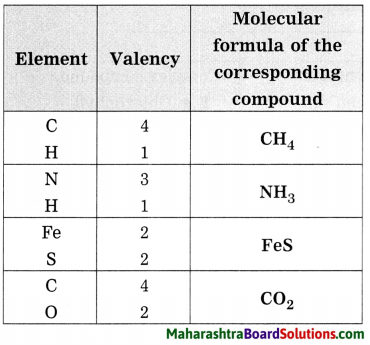Balbharti Maharashtra State Board Class 8 Science Solutions Chapter 6 Composition of Matter Notes, Textbook Exercise Important Questions and Answers.

Maharashtra State Board Class 8 Science Solutions Chapter 6 Composition of Matter

Class 8 Science Chapter 6 Composition of Matter Textbook Questions and Answers

1. Choose the appropriate option and rewrite the following statements:

Question a.
The intermolecular force is ……………. in the particles of solid.
(a) minimum
(b) moderate
(c) maximum
(d) indefinite
(c) maximum

Question b.
Solids retain their volume even when external pressure is applied. This property is called …………… .
(a) plasticity
(b) incompressibility
(c) fluidity
(d) elasticity
(d) elasticity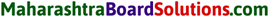Question c.
Matter is classified into the types mixture, compound and element, by applying the criterion …………… .
(a) states of matter
(b) phases of matters
(c) chemical composition of matter
(d) all of these
(c) chemical composition of matter

Question d.
Matter that contain two or more constituent substances is called ……… .
(a) mixture
(b) compound
(c) element
(d) metalloid
(a) mixture

Question e.
Milk is an example of type of matter called …………. .
(a) solution
(b) homogeneous mixture
(c) heterogeneous mixture
(d) suspension
(c) heterogeneous mixture

Question f.
Water, mercury and bromine are similar to each other, because the three are ……….. .
(a) liquids
(b) compounds
(c) nonmetals
(d) elements
(a) liquids

Question g.
Valency of carbon is 4 and that of oxygen is 2. From this, we understand that there are …………. chemical bond/bonds between the carbon atom and one oxygen atom in the compound- carbon dioxide.
(a) 1
(b) 2
(c) 3
(d) 4.
(b) 22. Identify the odd term out and explain.

Question a.
Gold, silver, copper, brass.
Brass. (Others are elements.)

Question b.
Hydrogen, hydrogen peroxide, carbon dioxide, water vapour.
Hydrogen. (Others are compounds.)

Question c.
Milk, lemon juice, carbon, steel.
Carbon. (Others are mixtures.)

Question d.
Water, mercury, bromine, petrol.
Petrol. (Others are inorganic compounds.)

Question e.
Sugar, salt, baking soda, blue vitriol.
Sugar. (Others are inorganic compounds.)Question f.
Hydrogen, sodium, potassium, carbon.
Carbon. (Others are monovalent elements)

Question a.
Plants synthesize glucose in sunlight with the help of chlorophyll from carbon dioxide and water and give away oxygen. Identify the four compounds in this process and name their types.
Photosynthesis: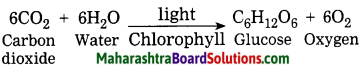Carbon dioxide, water, glucose, and Chlorophyll are compounds.
Types: Organic compounds: Glucose
Inorganic compounds: Carbon dioxide and water
Complex compounds: Chlorophyll.

Question b.
In one sample of brass, the following ingredients were found: copper (70%) and zinc (30%). Identify the solvent, solute and solution from these.
Brass is an alloy, it contains 70% copper and 30% zinc. The largest proportion is solvent, i.e. copper. The smaller proportion is solute, i.e. zinc. The solution is Brass.Question c.
Seawater tastes salty due to the dissolved salt. The salinity (the proportion of salts in water) of some water bodies Lonar lake – 7.9%, Pacific Ocean 3.5%, Mediterranean sea – 3.8%, 5 Dead sea – 33.7%. Explain two characteristics of mixtures from the above information.

1. The constituents of a mixture (the proportion of salts in water) do not combine chemically.
2. Their constituents are present in any proportion by weight.
3. The constituent of a mixture can be separated by a physical process.

4. Give two examples each.

Question a.
Liquid element.
Mercury (Hg), Bromine (Br2)

Question b.
Gaseous element.
Hydrogen (H2), Oxygen (O2).

Question c.
Solid element.
Iron (Fe), Copper (Cu), Silver (Ag).

Question d.
Homogeneous mixture.
Sea water, blue vitriol dissolved in water.

Question e.
Colloid.
Milk, blood.Question f.
Organic compound.
Glucose, urea.

Question g.
Complex compound.
Chlorophyll, Haemoglobin.

Question h.
Inorganic compound.
Soda, rust, limestone.

Question i.
Metalloid.
Silicon, arsenic.

Question j.
Element with valency 1.
Sodium (Na), potassium (K), chlorine (Cl).

Question l.
Element with valency 2.
Magnesium (Mg), Calcium (Ca).

5. Write the names and symbols of the constituent elements and identify their valencies from their molecular formulae given below:
KCl, HBr, MgBr2. K2O, NaH, CaCl2, CCl4, HI, H2S, Na2S, FeS, BaCl2.

Question a.
Write the names and symbols of the constituent elements and identify their valencies from their molecular formulae given below:
KCl, HBr, MgBr2, K2O, NaH, CaCl2, CCl4, HI, H2S, Na2S, FeS, BaCl2.

 Molecular formula Constituent element/Name symbol Valency 1. KCl Potassium (K) Chlorine (Cl) 1 1 2. HBr Hydrogen (H) Bromine (Br) 1 1 3. MgBr2 Magnesium (Mg) Bromine (Br) 2 1 4. K2O Potassium (K) Oxygen (O) 1 2 5. NaH Sodium (Na) Hydrogen (H) 1 1 6. CaCl2 Calcium (Ca) Chlorine (Cl) 2 1 7. CCl4 Carbon (C) Chlorine (Cl) 4 1 8. HI Hydrogen (H) Iodine (I) 1 1 9. H2S Hydrogen (H) Sulphur (S) 1 2 10. Na2S Sodium (Na) Sulphur (S) 1 2 11. FeS Iron (Fe) Sulphur (S) 2 2 12. BaCl2 Barium (Ba) Chlorine (Cl) 2 16. Chemical composition of some matter is given in the following table. Identify the main type of matter from them.

Question a.
Chemical composition of some matter is given in the following table. Identify the main type of matter from them.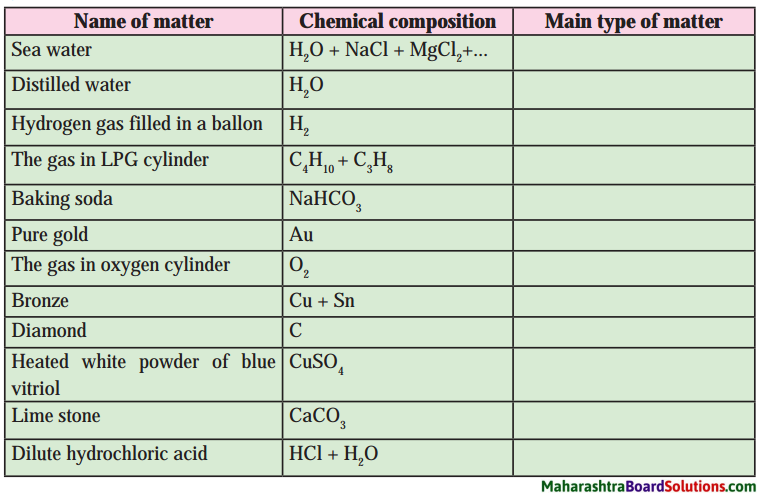Name of matter Chemical composition Main type of matter Sea water H2O + NaCl + MgCl2 +… Mixture Distilled water H2O Compound Hydrogen gas filled in a balloon H2 Element The gas in LPG cylinder C4H10 + C3H8 Mixture Baking soda NaHCO3 Compound Pure gold Au Element The gas in oxygen cylinder O2 Element Bronze Cu + Sn Mixture Diamond C Element Heated white powder of blue vitriol CuSO4 Compound Limestone CaCO3 Compound Dilute hydrochloric acid HCl + H2O Mixture7. Write scientific reason.

Question a.
Hydrogen is combustible, oxygen helps combustion, but water helps to extinguish fire.

1. Water is a compound of hydrogen and oxygen.
2. In a compound, the constituents do not retain their individual properties. Hence, hydrogen is combustible and oxygen helps combustion, but water is neither combustible nor supports combustion, it helps to extinguish fire.

Question b.
The constituent substances of a colloid cannot be separated by oridinary filtration.

1. A colloidal solution is heterogeneous.
2. The diameters of colloidal particles are of the order of 10-5 m.
3. The particles of a colloid can easily pass through a filter paper as the pore size of a filter paper is big. Hence, the constituents of a colloidal cannot be separated by filtration.

Question c.
Lemon sherbat has sweet, sour and salty taste and it can be poured in a glass.

1. Lemon sherbat is a mixture. It is made up of lemon juice, sugar, salt and water.
2. Formation of lemon sherbat does not involve any chemical reaction.
3. The constituents of sherbat retain their individual properties. Hence, lemon sherbat is sweet, sour and salty to taste and it can be poured in a glass.Question d.
A solid matter has the properties of definite shape and volume.

1. The forces among the constituent particles (atom/molecules) are called intermolecular forces.
2. In solids these forces are strong enough to keep the particles together in fixed positions, as a result solids have a definite shape and volume.

8. Deduce the molecular formulae of the compound obtained from the following pairs of elements by the cross multiplication method.

Question a.
C (Valency 4) & Cl (Valency 1)
Step 1: Write the symbols of the constituent elements.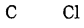Step 2: Write the valency below the respective elements.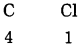Step 3: Cross multiply the valencies.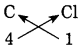The molecular formula: CCl4

Question b.
N (Valency 3) & H (Valency 1)
Step 1: Write the symbols of the constituent elements.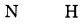Step 2: Write the valency below the respective elements.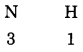Step 3: Cross multiply the valencies.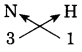The molecular formula: NH3Question c.
C (Valency 4) & O (Valency 2)
Step 1: Write the symbols of the constituent elements.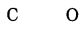Step 2: Write the valency below the respective elements.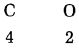Step 3: Cross multiply the valencies.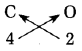The formula: C2O4
The number of constituent atoms in the final molecular formula should be the smallest possible whole number. Divide the formula C2O4 by suitable number.
Final molecular formula obtained by dividing by ‘2’.
Molecular formula: CO2

Question d.
Ca (Valency 2) & O (Valency 2)
Step 1: Write the symbols of the constituent elements.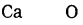Step 2: Write the valency below the respective elements.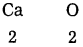Step 3: Cross multiply the valencies.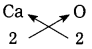The formula: Ca2O2
Divide the formula by suitable number ‘2’.
The molecular formula: CaO.Project:

Question a.
Collect the wrappers of ready-made foodstuff. Use the information given and prepare a chart of foodstuff and the ingredients in it. Procure the ingredients available. Discuss with friends and teacher, test the procured ingredients with combustion test under the supervision of your teacher. Thereby identify the ingredients as organic or inorganic.

Class 8 Science Chapter 6 Composition of Matter Additional Important Questions and Answers

Rewrite the sentences after filling the blanks:

Question 1.
………….. have no definite shape, but have a definite volume.
Liquids have no definite shape, but have a definite volume.

Question 2.
………… have neither a definite shape nor a definite volume.
Gases have neither a definite shape nor a definite volume.

Question 3.
The properties of a …………… are different than those of the constitute elements.
The properties of a compound are different than those of the constitute elements.Question 4.
The part of matter having uniform composition is called ………………. .
The part of matter having uniform composition is called phase.

Question 5.
The heterogeneous mixture of a liquid and a solid is called a ……………. .
The heterogeneous mixture of a liquid and a solid is called a suspension.

Question 6.
A colloid is a ………………. .
A colloid is a heterogeneous mixture.

Question 7.
Bronze contains atoms of the elements ……………. and ………….. .
Bronze contains atoms of the elements copper and tin.

Question 8.
When all the components of a mixture form one phase, it is called ………….. .
When all the components of a mixture form one phase, it is called homogeneous mixture.

Question 9.
…………… is a mixture of water, lactose, fats and proteins.
Milk is a mixture of water, lactose, fats and proteins.Question 10.
The intermolecular force is …………….. in the gaseous state.
The intermolecular force is very weak in the gaseous state.

Choose the appropriate option and rewrite the following statements:

Question 1.
When a liquid is poured from one container to another, its shape may change, but its volume ………….. .
(a) increases
(b) decreases
(c) remains the same
(d) none of them
(c) remains the same

Question 2.
The property by which some solids maintain their shape even when subjected to external forces is called ………. .
(a) elasticity
(b) fluidity
(c) rigidity
(d) plasticity
(c) rigidityQuestion 3.
…………… is a complex compound.
(a) Glucose
(b) Blue vitriol
(c) Chlorophyll
(d) Soda
(c) Chlorophyll

Question 4.
………….. is a homogeneous mixture.
(a) Sand + water
(b) Flour + water
(c) Salt + water
(d) Oil + water
(c) Salt + water

State whether the following statements are True or False:

Question 1.
Liquids do not have a definite shape
True.

Question 2.
Gases have a definite shape and volume.
False. (Gases do not have shape and volume.)

Question 3.
The crystals of blue vitriol form a single phase.
True.

Question 4.
Water is a mixture.
False. (Water is a compound)

Question 5.
Milk is a colloid.
True.

Question 6.
A suspension does not scatters light.
True.

Question 7.
Copper sulphate is a mixture.
False. (Copper sulphate is a compound.)Question 8.
An atom forms as many chemical bonds with other atom as its valency.
True.

Question 9.
The strength of intermolecular forces is weak in the liquid state.
False. (The strength of intermolecular forces is moderate in the liquid state.)

Question 10.
All the gases present together constitute a single phase.
True.

Match the column:

Question 1.

 Column I Column II 1. Potassium dichromate a. Complex compound 2. Cyanocobalamine b. Element 3. Air c. Compound 4. Silver d. Mixture

 Column I Column II 1. Potassium dichromate c. Compound 2. Cyanocobalamine a. Complex compound 3. Air d. Mixture 4. Silver b. Element

Question 2.

 Column 1 Column II 1. Milk a. Alloy 2. Lemonade b. Colloid 3. Sand and water c. Solution 4. Brass d. Suspension

 Column 1 Column II 1. Milk b. Colloid 2. Lemonade c. Solution 3. Sand and water d. Suspension 4. Brass a. AlloyQuestion 3.

 Column I Column II 1. Sea water a. Solid in solid 2. Vinegar b. Solid in liquid 3. Air c. Liquid in liquid 4. Bronze d. Gas in liquid 5. Chlorinated water e. Gas in gas

 Column I Column II 1. Sea water b. Solid in liquid 2. Vinegar c. Liquid in liquid 3. Air e. Gas in gas 4. Bronze a. Solid in solid 5. Chlorinated water d. Gas in liquid

Answer the following questions in one sentence:

Question 1.
Why does a solid have definite volume ?
In solids intermolecular forces are strong enough to keep the particles together in fixed positions, as a result solids have definite volume.

Question 2.
Why does a liquid have indefinite shape?
In a liquid intermolecular forces are not strong enough to keep the particles together in fixed positions, as a result liquid has indefinite shape.Question 3.
Why does a gas have indefinite volume and shape?
In a gas intermolecular forces are very weak and the distance between the particles is very large, as a result gas has indefinite volume and shape.

Question 4.
What can you say about the distance between the neighbouring particles of solid?
The particles of solid are very close to each other and the distance between them is minimum.

Question 5.
What can you say about the distance between the constituent particles of gas?
The intermolecular force is very weak in gases. The distance between the particles of gas is very large. They move freely and occupy all the available space.

Question 6.
State whether the mixture of oil in water is a homogeneous or heterogeneous mixture.
The mixture of oil in water is a heterogeneous mixture.

Question 7.
State whether seawater is a homogeneous or heterogeneous mixture.
Seawater is a homogeneous mixture.Question 8.
Give two examples of liquid in liquid.
Examples of liquid in liquid:
Vinegar, dilute sulphuric acid.

Question 9.
Give two examples of gas in gas.
Examples of gas in gas: Air, cooking gas.

Question 10.
Give two examples of solid in solid.
Examples of solid in solid: Brass, stainless steel.

Question 11.
Give two examples of gas in liquid.
Examples of gas in liquid: Chlorinated water, hydrochloric acid.

Question 12.
Give molecular formulae of 1. potassium chloride, 2. sodium sulphide.

1. Molecular formula of potassium chloride: KCl
2. Molecular formula of sodium sulphide: Na2S.

Question 13.
State the molecular formula and number of hydrogen atoms in methane.
Molecular formula of methane: CH4
The number of hydrogen atoms in methane is 4.

Question 14.
Identify the valency of chlorine in
(i) KCl and (ii) CaCl2.
i. The valency of chlorine in KCl is 1.
ii. The valency of chlorine in CaCl2 is 1.

Question 1.
State two principal ways of classifying matter.
Two principal ways of classifying matter are:

1. On the basis of physical state as a solid, liquid or gas.
2. On the basis of chemical constitution as an element, compound or mixture.Question 2.
What are the different states of matter?
The different states of matter are solid, liquid and gas.

Question 3.
Define solid.
A substance which has a definite shape and volume is called a solid.

Question 4.
State any three characteristics of solids.

1. Solids have a definite shape and volume.
2. Most of the solids are rigid while some are plastic and some elastic.
3. Solids have practically negligible compressibility, i.e., their volume is not very much changed by external forces.

Question 5.
What is meant by rigidity of solids?
The property by which solids maintain their shapes when subjected to external forces is called rigidity.

Question 6.
What is meant by elasticity of solids?
Some solids undergo a change in their shapes and volume when subjected to external forces and regain their original shapes and volume on removal of the forces. This property of solids is called elasticity.Question 7.
What is meant by plasticity?
Some solids are deformed by external forces and do not regain their original shapes on removal of the forces. This property of solids is called plasticity.

Question 8.
Solids have a high density and negligible compressibility. Explain.

1. The forces among the constituent particles (atoms/molecules) are called intermolecular forces.
2. In solids these forces are strong enough to keep the particles together in fixed positions, as a result solids have a high density and negligible compressibility.

Question 9.
Define liquid.
A substance which does not have a definite shape, but has a definite volume is called a liquid.

Question 10.
State any three characteristics of liquids.

1. Liquids have a definite volume.
2. Liquids do not have a definite shape. They take the shape of the container in which they are stored.
3. When they spill on a plane surface, they spread and flow in the surrounding areas, i.e., they have fluidity.
4. They have very small compressibility.

Question 11.
What is meant by fluidity?
Liquids flow easily and change their shapes in response to external forces. This property of liquids is called fluidity. It is exhibited by gases also.Question 12.
Liquids have fluidity and they do not have a definite shape. Explain.
1. The forces among the constituent particles (atoms/molecules) are called intermolecular forces.
2. In liquids, the intermolecular forces are strong enough to keep the particles within the bulk in aggregation, but not strong enough to keep them in fixed positions. As a result, they flow easily and change shapes according to that of container.

Question 13.
Define gas.
A substance which has neither a definite shape nor a definite volume is called a gas.

Question 14.
State any three characteristics of gases.

1. Gases have neither a definite shape nor a definite volume.
2. They spread in all directions.
3. They can be compressed or expanded easily.
4. In gases intermolecular forces are very weak.

Question 15.
Gases have neither a definite shape nor a definite volume. Explain.

1. The forces among the constituent particles (atoms/molecules) are called intermolecular forces.
2. In gases the intermolecular forces are extremely weak. As a result, the molecules of a gas move freely and a gas occupies all the available space.Question 16.
Why are gases more compressible, than liquids?
In liquids the distances between the neighbouring molecules are less than those in the gases. The intermolecular forces are extremely weak in gases, but not so weak in liquids. Hence, gases are more compressible than liquids.

Question 17.
What is an element? Give two examples.
A type of matter composed of only one kind of substances where each smallest indivisible part of it has the same properties is called an element. It cannot be subdivided into simpler substances by any physical or chemical means.
Examples: Oxygen, nitrogen, silver.

Question 18.
Write any two characteristics of an element.

1. All the atoms/molecules of an element are alike.
2. Atoms/molecules of different elements are different.
3. An element cannot be decomposed into simple substances by any chemical reaction or simple physical process.

Question 19.
What is a compound? Give two examples.
A substance produced by a chemical reaction of two or more elements combined in a fixed proportion is called a compound.
Examples: Water, common salt, sugar.Question 20.
Write any two characteristics of a compound.

1. The proportion of constituent elements in a compound is constant.
2. The properties of a compound is different than those of the constituent elements.

Question 21.
What is a mixture? Give two examples.
When two or more elements or compounds which do not react chemically with each other are mixed in any proportion, a mixture is formed.
Examples: Air, steel, milk.

Question 22.
Write any two characteristics of a mixture.

1. The constituent molecules of mixture are different from each other and notoined by chemical bonds.
2. The proportion of constituent substances are retained in the mixture.
3. The constituents of a mixture can be separated by a physical process.

Question 23.
Classify the following into elements, compounds and mixtures:
Sugar, sodium, nitrogen, sodium carbonate, air, sulphuric acid, mercury, stainless steel, cement, potassium dichromate.

• Elements: Sodium, nitrogen, mercury.
• Compounds: Sugar, sodium carbonate, sulphuric acid, potassium dichromate.
• Mixtures: Air, stainless steel, cement.Question 24.
Write the composition of the following materials by means of chemical formulae and classify them accordingly.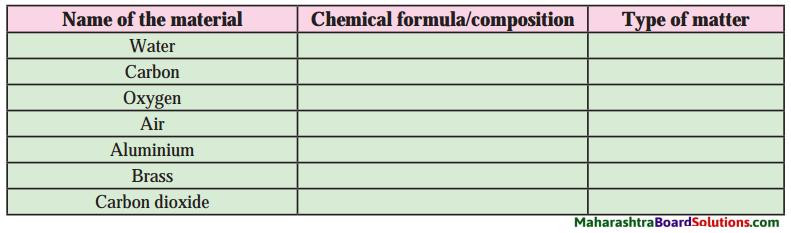Name of the material Chemical formulae/composition Type of matter Water H2O Compound Carbon C Element Oxygen O2 Element Air O2 + N2 + CO2 + other gases Mixture Aluminium Al Element Brass Cu + Zn Mixture Carbon dioxide CO2 Compound

Question 25.
What is meant by an organic compound? Give two examples.
The compound which when heated strongly gives black coloured carbon residue is called an organic compound or carbon compound.
Examples: Carbohydrates, proteins, hydrocarbons (petrol, cooking gas).Question 26.
What is meant by an inorganic compound? Give two examples.
Compounds which when heated strongly decomposes to give a residue behind are called inorganic compounds.
Examples: Common salt, rust, blue vitriol, limestone.

Question 27.
What is meant by complex compounds? Give two examples.
The molecules of compounds which have a complex structure formed by many atoms and in the centre of this structure metal atoms are also included are called complex compounds.
Examples: Haemoglobin, chlorophyll.

Question 28.
Classify the following compounds into organic compounds, inorganic compounds and complex compounds.
Petrol, common salt, haemoglobin, blue vitriol, cyanocobalamine, cooking gas.

• Organic compounds: Petrol, cooking gas
• Inorganic compounds: Common salt, blue vitriol.
• Complex compounds: Cyano cobalamine, haemoglobin.

Question 29.
What is a phase?
The part of matter with uniform composition is called a phase.

Question 29.
What is a homogeneous mixture? Give two examples.
When all the components of a mixture form one phase, it is called homogeneous mixture.
Example: Solution of blue vitriol in water, solution of sugar in water.Question 30.
What is heterogeneous mixture? Give two examples.
When the components of a mixture are distributed into two or more phases, it is called a heterogeneous mixture.
Examples: Oil and water, sand and water.

Question 31.
Define solution or What is meant by a solution? Give two examples.
A homogeneous mixture of two or more substances is called a solution.
Examples: Seawater, sugar, syrup.

Question 32.
What is meant by a solvent?
OR
Define solvent. Give two examples.
The component which is present in largest proportion in a solution is called a solvent.
Examples:

1. In sea water, water is the solvent.
2. In tincture iodine, alcohol is the solvent.

Question 33.
What is meant by a solute?
OR
Define solute. Give two examples.
The component of a solution which is present in smaller proportion than the solvent is called a solute.
Examples:

1. In sea water, salt is a solute.
2. When a small of amount of sugar is dissolved in water, sugar is the solute in the solution.

Question 34.
Define suspension.
OR
What is meant by a suspension?
A heterogeneous mixture of a liquid and a solid is called a suspension. The diameter of the solid particles in a suspension is larger than 10-4 m.Question 35.
Define colloid.
A heterogeneous mixture in which the particles cannot be seen with a naked eye is called a colloid. The diameter of colloidal particles is around 10-5 m.

Question 36.
State the characteristics of a solution.

1. A solution is clear and transparent, it passes through a filter paper.
2. The particles are not visible to the naked eye.
3. A light can easily pass through it without scattering.
4. A solution is homogeneous mixture.

Question 37.
State the characteristics of a suspension.

1. In suspension, the solute particles do not dissolve but remain suspended in it. The liquid and solid components of suspension are separated by filtration.
2. The particles are visible to the naked eye.
3. The particles in suspension are of very small size and scatter a beam of light passing through it.
4. It is heterogeneous in nature.

Question 38.
State the characteristics of a colloid.

1. In a colloid, the particles are spread uniformly throughout the solution.
2. The size of the particles is less than that of the particles in a suspension.
3. The particles are not visible to the naked eye.
4. The particles scatter a beam of light.
5. A colloidal solution appears to be homogeneous, but actually it is heterogeneous. ‘Question 39.
Give two examples of each of the following:

1. Phase
2. Homogeneous matter
3. Heterogeneous matter
4. Homogeneous mixture
5. Heterogeneous mixture
6. Solution
7. Suspension
8. Colloid.

1. Phase: (i) Solid: Sodium chloride, blue vitriol, sand, (ii) Liquid: Water, alcohol, (iii) Gas: Chlorine, ammonia.
2. Homogeneous matter: Blue vitriol (solid), a solution of blue vitriol in water (a solid in a liquid), chlorinated water (a gas in a liquid).
3. Heterogeneous matter: Sand and water, sand and alcohol, blue vitriol and sand.
4. Homogeneous mixture: A solution of sodium chloride in water, air, a mixture of water and alcohol.
5. Heterogeneous mixture: A mixture of sand and blue vitriol, sodium chloride and iron filings, oil and water.
6. Solution: Seawater, a solution of sugar in water, a solution of blue vitriol in water.
7. Suspension: A mixture of sand and water, sand and alcohol, muddy water, limestone in water.
8. Colloid: Milk, milk of magnesia, blood, printing ink.

Question 40.
State the different types of solutions. Give one example.
The different types of solutions:

• Liquid in liquid. Examples: Vinegar, dilute sulphuric acid.
• Gas in gas. Example: Air.
• Solid in solid. Examples: Brass, stainless steel, bronze.
• Gas in liquid. Examples: Chlorinated water.Question 41.
Classify the following into homogeneous solution and heterogeneous solution?
Soda water, potassium permanganate solution, milk, alcohol in water, ink solution, wheat flour in water.

• Homogeneous solution: Soda water, potassium permanganate solution, alcohol in water.
• Heterogeneous solution: Milk, ink solution, wheat flour in water.

Question 42.
Classify the following into solution, suspension and colloids:
Milk, sand in water, lemonade, smoke, oxygen in water.

• Solution: Lemonade, oxygen in water.
• Suspension: Sand in water.
• Colloids: Milk, smoke.

Question 43.
What is meant by molecular formula?
The number of atom of each of the constituent elements present in one molecule of a compound is called molecular formula.

Question 44.
What is meant by valency of an element?
The capacity of an element to combine with another element is known as valency.

Question 45.
Deduce the molecular formulae of the compound obtained from the following pairs of elements by the cross multiplication method.
Question a.
H (Valency 1) and O (Valency 2)
Step 1: Write the symbols of the constituent elements.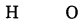Step 2: Write the valency below the respective elements.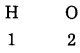Step 3: Cross multiply the valencies.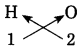∴ The molecular formula: H2O.Question b.
Fe (Valency 2) and S (Valency 2)
Step 1: Write the symbols of the constituent elements.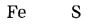Step 2: Write the valency below the respective elements.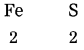Step 3: Cross multiply the valencies.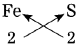The molecular formula: FeS

Question c.
The valencies of the atom H, O and N are 1, 2 and 3 respectively. The molecular formulae of these gaseous elements are H2, O2 and N2 respectively. How many chemical bonds are there in each of these molecules?
Chemical bond in H2 is one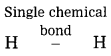Chemical bond in O2 are two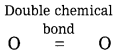Chemical bond in N2 are three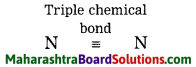Give scientific reasons:

Question 1.
Hydrogen is an element.

1. Hydrogen has only one kind of atoms.
2. Hydrogen cannot be further divided into new substances by any physical or chemical means. Therefore, hydrogen is an element.

Question 2.
Water is a compound.

1. The composition of water is same throughout i.e., the elements hydrogen and oxygen whose atoms are combined in the ratio by weight is always 1 : 8.
2. The properties of water are totally different from those of its constituents, i.e. hydrogen and I oxygen. Hence, water is a compound.?Question 3.
Air is a mixture.

1. Air contains nitrogen, oxygen and other gases.
2. These gases retain their original properties. They do not combine with each other. Hence, air is a mixture.

Question 4.
A potassium permanganate solution in water is a homogeneous mixture, while a mixture of oil and water is a heterogeneous mixture.

1. In a potassium permanganate solution in water the constituents, i.e. potassium permanganate and water are uniformly mixed throughout.
2. The properties and composition of a homogeneous mixture are the same throughout the mixture. Hence, potassium permanganate solution is a homogeneous mixture.
3. In a mixture of oil and water, the constituents are not uniformly mixed throughout the mixture.
4. The properties and composition of a heterogeneous mixture are not the same throughout the mixture. Hence, a mixture of oil and water is a heterogeneous mixture.

Question 5.
Milk is a colloid.

1. In milk, the particles are spread uniformly throughout and due to very small size of the particles, milk appears to be homogeneous.
2. But milk is a colloid solution and it is a heterogeneous mixture. Here, milk is liquid particles of proteins and fats having a diameter around 10-5 m dispersed in the aqueous medium. Hence, a milk is a colloid.

Distinguish between the following:

Question 1.

 Solids Liquids 1.  Solids have a definite shape. 1. Liquids have no definite shape. 2.  They have very low compressibility. 2. They have moderate compressibility. 3. In solids, the intermolecular forces are very strong. E.g. iron, stone, salt. 3. In liquids, the intermolecular forces are moderately strong. E.g., water, alcohol, mercury.Question 2.
Liquids and Gases:

 Liquids Gases 1. Liquids have a definite volume. 1. Gases do not have a definite volume. 2. They have moderate compressibility. 2. They have high compressibility. 3. In liquids, the intermolecular forces are moderately strong. E.g. water, alcohol, mercury. 3. In gases, the intermolecular forces are very weak. E.g. oxygen, air.

Question 3.
Solids and Gases:

 Solids Gases 1. Solids have a definite shape and volume. 1. Gases have neither a definite shape nor a definite volume. 2. They have incompressibility. 2. They have high compressibility 3. In solids, the intermolecular forces are very strong. E.g. iron, stone. 3. In gases, the intermolecular forces are very weak. E.g. hydrogen, oxygen.

Question 4.
Element and Compound:

 Element Compound 1. An element consists of only one kind of substance. 1. A compound is formed from two or more elements. 2. An element cannot be further divided into simpler substances by any physical or chemical means. E.g. iron, copper, oxygen. 2. A compound can be further divided into simple substances by chemical means. E.g. common salt, sugar, water.Question 5.
Compound and mixture:

 Compound Mixture 1. A compound is made of two or more elements chemically combined in a fixed proportion. 1. A mixture is obtained by just mixing two or more substances in any proportion. 2. A compound can be further subdivided into simpler substances by chemical means only. 2. A mixture can be further subdivided into simpler substances by simple physical processes. 3. The properties of a compound are entirely different from the properties of its constituents. E.g. common salt, sugar, water. 3. The constituents of a mixture retain their original properties. E.g. air, seawater.

Question 6.
Colloidals and Suspension:

 Colloidals Suspension 1. The particles in a colloidal solution can pass through a filter paper. 1. The particles in a suspension cannot pass through a filter paper. 2. The colloidal particles cannot be seen with a naked eye. 2. The suspended particles can be seen with a naked eye. 3. The diameter of solid particles is around 10-5 m 3. The diameter of the solid particles is larger than 10-4 m.

Activity-based questions:

Activity 1:
Material: Mustard seeds
Take some mustard seeds in a transparent plastic jar. Thread a long thread at the centre of a big balloon by means of a needle and tie it tight. Stretch this rubber diaphragm and fix it on the mouth of the jar by means or a rubber band. Pull the diaphragm up and down with the help of the thread first slowly, then with moderate force and then vigorously. Record your observation in the following table.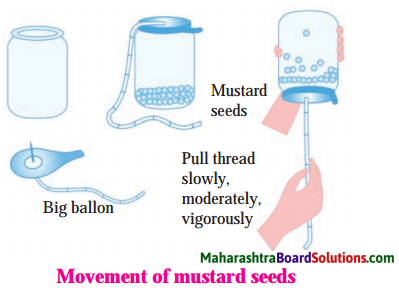Question 1.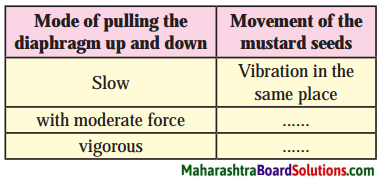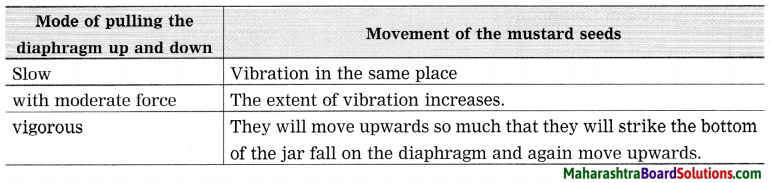Activity 2:
Apparatus: Hammer, sand paper
Material: Iron nail, copper wire, aluminium wire, coal.
Take the following objects: iron nail/sheet, copper wire, aluminium wire, a piece of coal. Rub each object on a fresh surface of sandpaper and observe. Hammer each object with force. (Take care not to hurt yourself.) Record your observations in the following table.
The objects in the activity are made of the elements iron (Fe), copper (Cu), aluminium (Al) and carbon (C); respectively. Fill the following table on the basis of the observations obtained on doing the above two tests on each of the objects.

Question 1.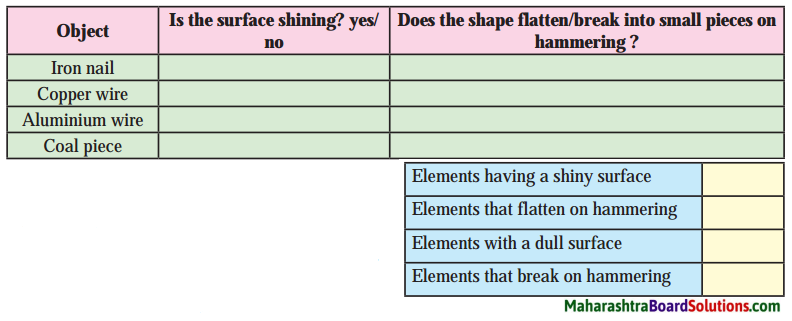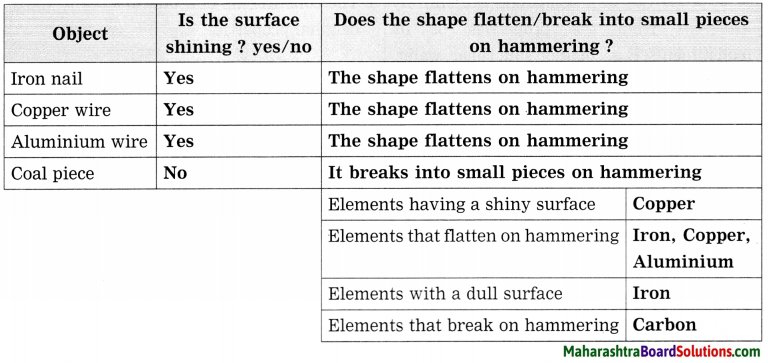Activity 3:
Apparatus: Evaporating dish, tripod stand, burner, etc.
Chemicals: Camphor, washing soda, blue vitriol, sugar, glucose, urea.
Keep the evaporating dish on the tripod stand. Take some camphor in the evaporating dish. Heat the camphor in the dish strongly with the help of a burner. Find out what remains behind in the evaporating dish. Repeat the above procedure using limestone, washing soda, blue vitriol, sugar, glucose and urea instead of camphor. Record your observation in the following table. (Do this activity carefully under the supervision of your teacher, as some of the powders may catch fire.)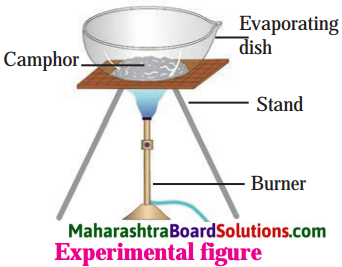Question 1.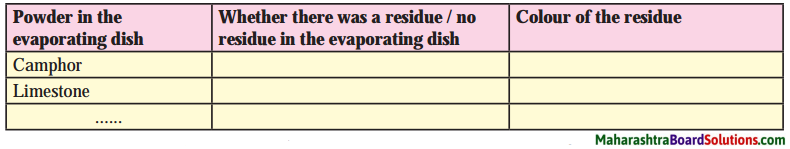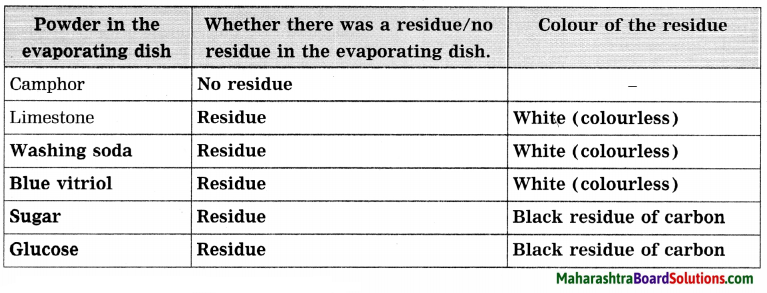Activity 4: (Textbook page 43)
Apparatus: Beakers, rods
Chemicals: Blue vitriol, sand, water, etc.
Take three beakers. Take a little sand and water in the first beaker. Take some crystals of blue vitriol and water in the second beaker. Take some blue vitriol and sand in the third beaker. Stir the materials in all the three beakers and observe. Record your observation in the following table.

Question 1.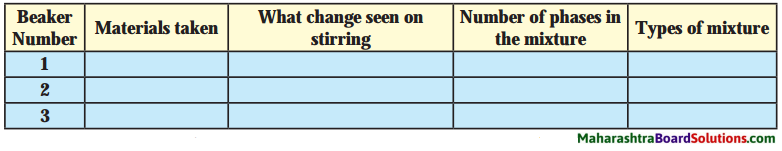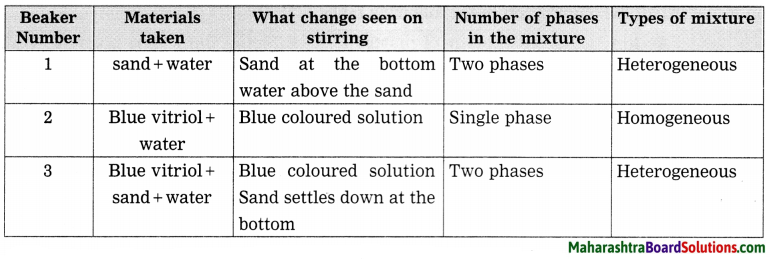Question 2.
In the above activity after stirring a homogeneous mixture is formed in only one beaker? Which is that?
The homogeneous mixture is formed in the second beaker. It is a solution of blue vitriol.Activity 5:
Apparatus: Beaker, conical flask, funnel, filter paper
Chemicals: Common salt, saw dust, milk.
Procedure: Take three beakers. Take 10 g common salt in the first beaker, 10 g saw dust in the second beaker and 10 ml milk in the third beaker. Add 100 ml water to all the three beakers and stir. Which of the mixtures shows separate water phase ? Place the three beakers in front of a vertically held paper and pass a laser beam through the beakers from the opposite side. (Use the laser beam under the guidance of teacher.) At the same time observe what appears on the paper in front of the beaker. Also look at the beaker from the side. Arrange three filtration assemblies using conical flask, funnel and filter paper for doing filtration. Stir the mixtures in the three beakers and carry out filtration. Record all the observations in the following table.

Question 1.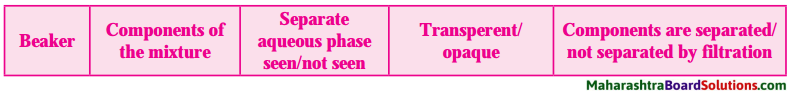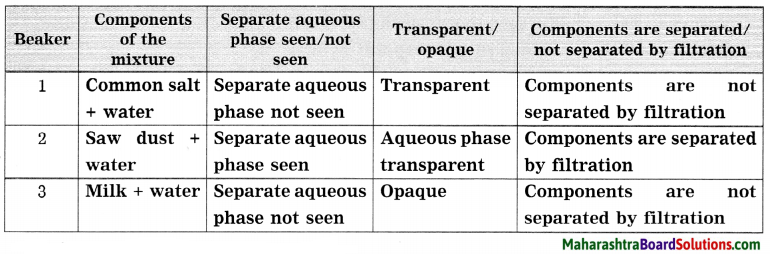Activity 6: (Textbook page 44)
Apparatus: Horseshoe magnet, evaporating dish, burner. Chemicals: Iron filings, sulphur.
Procedure: Take two evaporating dishes. Take 7 g iron filings in the first dish and 4 g sulphur in the second. Take a horseshoe magnet near the matter in both the dishes and observe. Transfer the entire iron filings from the first dish to the second, stir with a glass rod and observe by taking the horseshoe magnet near the matter. Also observe the colour of the matter. Now heat the matter in the second dish for a while and let it cool. Observe the colour change, if any in the matter and observe whether there is any effect of the horseshoe magnet on it. Record all the observation in the following table.

Question 1.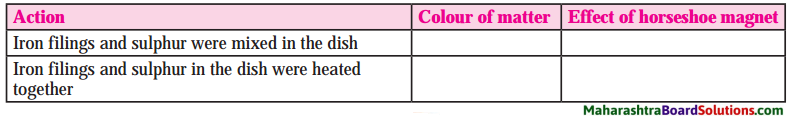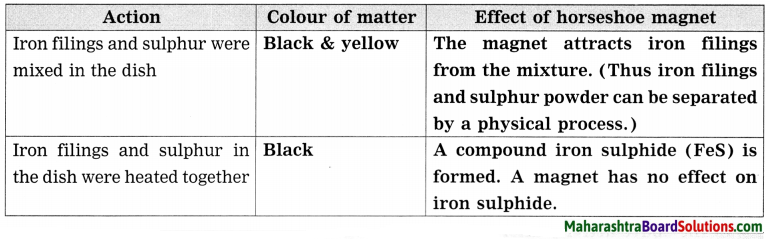Complete the following:

Question 1.
Complete the table: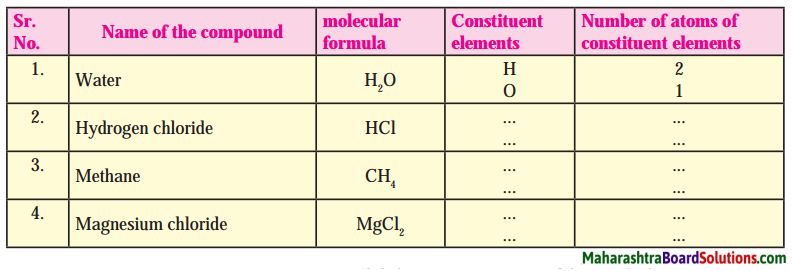Molecular formulae of some compounds are given in the following table. Use these to fill in the gaps in the table.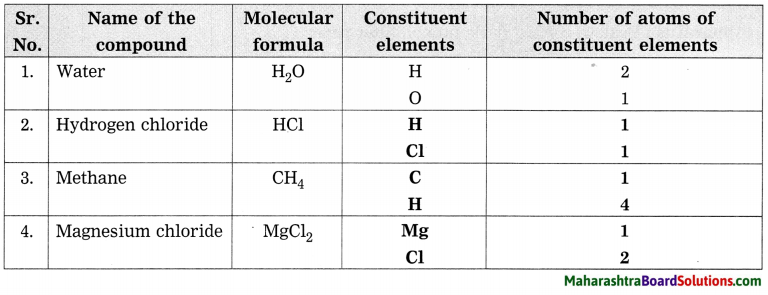Question 2.
Complete the table: